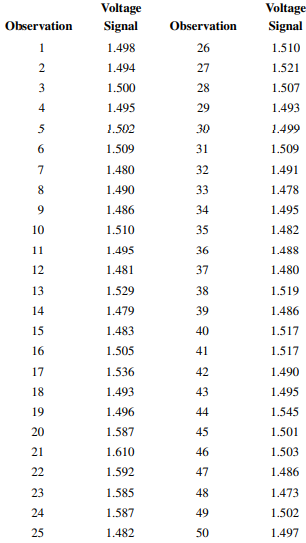×
Get Full Access to Applied Statistics And Probability For Engineers - 7 Edition - Chapter 15 - Problem 15.s29
Get Full Access to Applied Statistics And Probability For Engineers - 7 Edition - Chapter 15 - Problem 15.s29

×ISBN: 9781119409533 2045

## Solution for problem 15.S29 Chapter 15

Applied Statistics and Probability for Engineers | 7th Edition

• Textbook Solutions
• 2901 Step-by-step solutions solved by professors and subject experts
• Get 24/7 help from StudySoup virtual teaching assistantsApplied Statistics and Probability for Engineers | 7th Edition

4 5 1 256 Reviews
24
1
Problem 15.S29

An article in Microelectronics Reliability [“Advanced Electronic Prognostics through System Telemetry and Pattern Recognition Methods” (2007, Vol. 47(12), pp. 1865–1873)] presented an example of electronic prognostics (a technique to detect faults in order to decrease the system downtime and the number of unplanned repairs in high-reliability and high-availability systems). Voltage signals from enterprise servers were monitored over time. The measurements are provided in the following table.a. Using all the data, compute trial control limits for individual observations and moving-range charts. Construct the chart and plot the data. Determine whether the process is in statistical control. If not, assume that assignable causes can be found to eliminate these samples and revise the control limits

b. Estimate the process mean and standard deviation for the in-control process.

c. The report in the article assumed that the signal is normally distributed with a mean of 1.5 V and a standard deviation of 0.02 V. Do your results in part (b) support this assumption?

Step-by-Step Solution:
Step 1 of 3

Statistics 401 Notes Statistics is about collecting data, organizing it, summarization, and then analyzing it. Stats  Collecting o Ex: Surveys  Organization o Grouping the data using graphs or charts  Summarization o Finding the average- median, mean, mode o (center and spread of data- Ex: Standard deviation) o The lesser spread of data (smaller range) = a more reliable source because it is more consistent.  Ex: Data with outcomes of 30-70 (40 range) is MORE reliable than a range from 0-100 (100 range) o Population is the set of all units under study  Population is represented by a sample of these units (random sample).  Analyzing o Based on the sample drawn, you are able to make a conclusion about the population. Variable  Numerical (Quantitative) is when the data is measurable or countable o Ex: weight, age, height  Discrete: specific pattern that has specific values  Continuous: data that varies over an interval (infinite amount of answer between an interval)  Categorical (Qualitative) is when the data is NOT measurable o Ex: race or gender Q: How to tell which is numerical or categorical A: Finding the average must make sense. Ex: Finding the average weight makes sense because you can have an average weight of 100 lbs and that data can be used. Or, finding

Step 2 of 3

Step 3 of 3

## Discover and learn what students are asking

Statistics: Informed Decisions Using Data : Goodness-of-Fit Test
?Explain why chi-square goodness-of-fit tests are always right tailed.

Unlock Textbook Solution